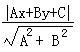• 编程求直线两点式方程 已知直线上的两点A1(X1,Y1) A2(X2,Y2)， A1、A2两点不重合。 则直线的一般方程AX+BY+C=0中： A = Y2 - Y1 B = X1 - X2 C = X2*Y1 - X1*Y2 即(Y2 - Y1)X + (X1 - X2)Y + ( X2*Y1 - X1*Y2) =...
编程求直线的两点式方程
已知直线上的两点A1(X1,Y1)   A2(X2,Y2)， A1、A2两点不重合。
则直线的一般式方程AX+BY+C=0中：
A = Y2 - Y1
B = X1 - X2
C = X2*Y1 - X1*Y2
即(Y2 - Y1)X + (X1 - X2)Y + ( X2*Y1 - X1*Y2) = 0
若对于一般式的变体AX+BY=C’：
A = Y2 - Y1
B = X1 - X2
C = X1*Y2 - X2*Y1
即(Y2 - Y1)X+(X1 - X2)Y = X1*Y2 - X2*Y1
*如果需要直接通过 A * x + B * y > C’ or A * x + B * y < C’ 的方法判断一个点是否在一条直线的上/下方，则需要保证求出来的B不为负。例如若A=8，B=-9，C=64，则需要将直线方程两边同时加负号，改为A=-8，B=9，C=-64。
*这种方法计算出直线方程时不需额外考虑斜率为0的特殊情况。因为斜率为0时B=0。


展开全文• 已知直线上的两点P1(X1,Y1) P2(X2,Y2)， P1 P2两点不重合。 对于AX+BY+C=0： 当x1=x2时，直线方程为x-x1=0 当y1=y2时，直线方程为y-y1=0 当x1≠x2，y1≠y2时，直线的斜率k=(y2-y1)/(x2-x1) 故直线方程为y-y1=...
已知直线上的两点P1(X1,Y1)  P2(X2,Y2)， P1 P2两点不重合。

对于AX+BY+C=0：

当x1=x2时，直线方程为x-x1=0

当y1=y2时，直线方程为y-y1=0

当x1≠x2，y1≠y2时，直线的斜率k=(y2-y1)/(x2-x1)

故直线方程为y-y1=(y2-y1)/(x2-x1)×(x-x1)

即x2y-x1y-x2y1+x1y1=(y2-y1)x-x1(y2-y1)

即(y2-y1)x-(x2-x1)y-x1(y2-y1)+(x2-x1)y1=0

即(y2-y1)x+(x1-x2)y+x2y1-x1y2=0 ①

可以发现，当x1=x2或y1=y2时，①式仍然成立。所以直线AX+BY+C=0的一般式方程就是：

A = Y2 - Y1

B = X1 - X2

C = X2*Y1 - X1*Y2

点到直线的距离公式：展开全文• 问题：分别已知两直线上的两点直线交点，给出解析解。已知直线l1有两点(x1, y1), (x2, y2)，直线l2上有两点(x3, y3), (x4, y4)，l1, l2的交点(x0, y0)。（用x1, x2, x3, x4, y1, y2, y3, y4来表示x0, y0）
分别已知两直线上的两点，求两直线交点

求两直线的交点是初中数学的简单问题了，在直角坐标系中直线有很多种表示方式。同时我们知道两点确定一条直线，已知两点坐标自然能求出直线坐标，已知两直线坐标自然能求出两直线交点。

问题：分别已知两直线上的两点，求两直线交点，给出解析解。已知直线 $l_1$ 上有两点 $(x_1, y_1), (x_2, y_2)$ ，直线 $l_2$ 上有两点 $(x_3, y_3), (x_4, y_4)$ ，求 $l_1, l_2$ 的交点 $(x_0, y_0)$ 。（用 $x_1, x_2, x_3, x_4, y_1, y_2, y_3, y_4$ 来表示 $x_0, y_0$）

本篇摒弃一切奇技淫巧，使用最简单的原理暴力计算得到无需分类讨论的通用结果。原理简单但推导过程繁杂丑陋，数学方面有洁癖的朋友可以跳过推导直接取用结果。

原理：$(x_0, y_0),(x_1, y_1), (x_2, y_2)$ 共线，列出共线方程（方程①）；$(x_0, y_0),(x_3, y_3), (x_4, y_4)$ 共线，列出共线方程（方程②），两个方程解两个未知数，利用轮换对称原理化简计算得到答案。

$(x_0, y_0),(x_1, y_1), (x_2, y_2)$ 共线，列出共线方程①：
$(y_0-y_1)\times(x_0-x_2) = (y_0-y_2) \times (x_0-x_1)$
$(x_0, y_0),(x_3, y_4), (x_3, y_4)$ 共线，列出共线方程②：（只需要上面方程的脚标1全部换成3，2全部换成4）
$(y_0-y_3)\times(x_0-x_4) = (y_0-y_4) \times (x_0-x_3)$
方程①展开得到方程(3)：
$x_0y_0-x_0y_1-x_2y_0+x_2y_1=x_0y_0-x_1y_0-x_0y_2+x_1y_2......(3)$
方程(3)消去二次项，再合并未知数前面的系数，得到方程(4)；由方程②经过同样的过程可以推得方程(5)，但实际上只需要将方程(4)中脚标1全部换成3，2全部换成4即可得到方程(5)：
$(x_1-x_2)y_0+x_2y_1=(y_1-y_2)x_0+x_1y_2......(4)\\(x_3-x_4)y_0+x_4y_3=(y_3-y_4)x_0+x_3y_4......(5)$
至此问题变成了解出方程(4)和方程(5)组成的二元一次方程组。尝试采用消元的方式解出 $x_0$ ，给方程(4)两边同时乘 $(x_3-x_4)$ 得到方程(6)，给方程(5)两边同时乘 $(x_1-x_2)$ 得到方程(7)：
$(x_1-x_2)(x_3-x_4)y_0+(x_3-x_4)x_2y_1=(x_3-x_4)(y_1-y_2)x_0+(x_3-x_4)x_1y_2......(6)\\(x_1-x_2)(x_3-x_4)y_0+(x_1-x_2)x_4y_3=(x_1-x_2)(y_3-y_4)x_0+(x_1-x_2)x_3y_4......(7)$
用方程(6)减去方程(7)得到方程(8)，即可得到 $x_0$ 的解：
$(x_3-x_4)(x_2y_1-x_1y_2)-(x_1-x_2)(x_4y_3-x_3y_4)=((x_3-x_4)(y_1-y_2)-(x_1-x_2)(y_3-y_4))x_0...(8)$
由于轮换对称特性，将 $x_0$ 表达式中的x全部换为y，y全部换为x即可得到 $y_0$ 的解。

最终的解是：
$x_0=\frac{(x_3-x_4)(x_2y_1-x_1y_2)-(x_1-x_2)(x_4y_3-x_3y_4)}{(x_3-x_4)(y_1-y_2)-(x_1-x_2)(y_3-y_4)}$
$y_0=\frac{(y_3-y_4)(y_2x_1-y_1x_2)-(y_1-y_2)(y_4x_3-y_3x_4)}{(y_3-y_4)(x_1-x_2)-(y_1-y_2)(x_3-x_4)}$
当二者的分母为0时，两直线平行，没有交点。

为了方便各位在代码中搬运，再给出如下形式：
x0 = ((x3-x4) * (x2*y1 - x1*y2) - (x1-x2) * (x4*y3 - x3*y4)) / ((x3-x4) * (y1-y2) - (x1-x2) * (y3-y4));
y0 = ((y3-y4) * (y2*x1 - y1*x2) - (y1-y2) * (y4*x3 - y3*x4)) / ((y3-y4) * (x1-x2) - (y1-y2) * (x3-x4));

为了方便各位以数组的形式在代码中搬运，再给出如下形式：
x0 = ((x - x) * (x * y - x * y) - (x - x) * (x * y - x * y)) / ((x - x) * (y - y) - (x - x) * (y - y));
y0 = ((y - y) * (y * x - y * x) - (y - y) * (y * x - y * x)) / ((y - y) * (x - x) - (y - y) * (x - x));



展开全文算法
• //直线过P1,P2两点，P0为直线外一点，P0到直线的距离和P0在直线上的垂足void HelloWorld::initPoint(cocos2d::CCPoint point0,cocos2d::CCPoint point1,cocos2d::CCPoint point2){ P1 = point1; //直线上的第一个...
//直线过P1,P2两点，P0为直线外一点，求P0到直线的距离和P0在直线上的垂足void HelloWorld::initPoint(cocos2d::CCPoint point0,cocos2d::CCPoint point1,cocos2d::CCPoint point2){ P1 = point1; //直线上的第一个点 P2 = point2; //直线上的第二个点 P0 = point0; //直线外的一点}//得到点到直线的距离float HelloWorld::getLenthPointToLine(){ A = P2.y - P1.y; B = P1.x - P2.x; C = P1.y*(P2.x - P1.x) - P1.x*(P2.y - P1.y);//直线为Ax+By+C=0 CCLog("A = %f,B = %f,C = %f",A,B,C);
d = fabsf(A*P0.x+B*P0.y+C)/sqrt(A*A+B*B); CCLog("d = %f",d); return d;}//得到点在直线上的垂足cocos2d::CCPoint HelloWorld::getPointToLineChuiZu(){ float D = A*P0.y - B*P0.x; float xd = -(B*D + A*C)/(B*B + A*A);
float yd; if (B == 0) {  yd = P0.y; }else{  yd = -(A*xd+C)/B; }
CCLog("c(%f,%f)",xd,yd);
return ccp(xd,yd);} 
展开全文•  平面上有n个,你要找一条直线,使得所有都是直线的同一侧(可以在直线上),且所有直线的距离和最小. 问你所有直线的距离和最小是多少(要求输出平均值)? 分析:  首先如果存在这样的直线,那么该直线肯定...algorithm 算法 计算几何
• 给定个整点的坐标，它们所在直线的函数解析式（一次函数）。 输入格式 输入共行。 第一行有个整数x1,y1。表示第一个整点的坐标为(x1,y1) 第二行有个整数x2,y2。表示第二个整点的坐标为(x2,y2) 输出格式 ...
• (一)点斜式已知直线l的斜率是k,并且经过点P1(x1,y1) 直线方程是y-y1=k(x-x1) ...(二)两点式：已知直线l上的两点P1(x1,y1)、P2(x2,y2),(x1≠x2) 直线方程是(y-y1)/(y2-y1)=(x-x1)/(x2-x1) 也要注意两...
• 给定个整点的坐标，它们所在直线的函数解析式（一次函数）。 输入输出格式 输入格式： 输入共行。 第一行有个整数x1,y1。表示第一个整点的坐标为(x1,y1) 第二行有个整数x2,y2。表示第二个整点的...
• 设有两空间线段 Ls，其起点、终点坐标为s0、s1，方向向量u⃗ =s1−s0 Lt，其起点、终点坐标为t0、t1，方向向量v⃗ =t1−t0 ...设最短距离两点分别为sj、tj，则有 sj=s0+sc⋅u⃗ tj=t0+sc⋅v⃗ 其...
• 已知图像中线段的另外一个到这个的垂足。 一、垂足公式 已知直线一般方程时： 设已知直线外一点坐标为（x0,y0）（x0,y0）（x0,y0）,垂足坐标为（x,y）（x,y）（x,y），因为垂线与直线垂直，垂线...
• js 实现 通过两点坐标计算直线距离! 值得下载看看!资源免费,大家分享!!js
• 用于拟合样条函数的数据：x f ( x)3.0 2.54.5 1.07.0 2.59.0 0.5 我们知道在平面上确定一条直线，三个确定一条抛物线（假设曲线的类型是抛物线），那么现在有四个，我们很自然的会想到，既然确定...
• MATLAB 已知两点坐标，求解直线方程代码 x1=input(‘请输入x1=’); y1=input(‘请输入y1=’); x2=input(‘请输入x2=’); y2=input(‘请输入y2=’); if (x1x2)&amp;&amp;(y1y2) disp(‘请输入两个不同的点’)...
• 高中数学必修二平面解析几何重点介绍两直线的位置关系基础知识和易误点，并用平面解析几何两直线3个经典习题和2017年高考试题归纳与整理。 一、 基础知识 1、 两直线的平行、垂直与其斜率的关系 2.直线的交点 ...高中数学
• §1 直线方程 ...1．直线的斜率 ...2．斜截 ... y=kx+b 中k为直线的斜率， ...4．截距 ... 中a,b分别为直线的横截距和纵截距. ...5．两点式     6．一般  Ax+By+C=0 中A,B不同时
• 最近老板让写一段空间匹配的代码， 其中涉及到空间两直线之间的距离，写起来满费劲的， 这里做一个记录。 2. 处理思路 空间两直线之间的位置关系主要可以分为： 重合， 平行， 相交， 异面。 2.1...
• //如果两个y差值相乘为0，则说明两点相交 } } 思路 1, 使用区间的方式来判断交点的位置，避免了很多运算，节约了资源．(参考0-1000猜数字的游戏) 2, 斜率之于直线/一次方程/线性，相当于导数之于曲线/...
• §1 空间直线的方向 1. 方向角  通过原点O的直线OM与三条坐标轴的夹角α，β，γ称为该直线的方向角 即: α=∠MOX，β=∠MOY，γ=∠MOZ 2. 方向余弦 直线方向角的余弦称为方向余弦。 l=cosα=x/ρ, m=...平面方程
• 使用C++求直线直线、圆、矩形交点坐标几何类的编写圆矩形直线总结 几何类的编写 圆 没什么特别的，利用了圆的标准公式来表示圆 class Circle { private: double x_center_param; /* 圆心的 x 坐标值 */ double y...
• §4 空间中点到直线、平面的距离 1.到平面的距离 1o 设平面P的法线方程为:   则M(xo ,yo ,zo)到平面P的距离为: ...设直线L的对称方程为:   (直线过M1(x1 , y1 , z1),方向数为p,平面之间的关系
• 已知直线两点求直线的一般方程 一般方程在计算机领域的重要性 常用的直线方程有一般 点斜式 截距 斜截 两点式等等。除了一般方程，它们要么不能支持所有情况下的直线（比如跟坐标轴垂直或者...
• 如下图所示，在直线L上取两点与，则得到向量，与构成向量，根据下计算得到两向量夹角。那么到直线L的距离为 方法二： 使用向量叉乘得到，如下图所示： 具体参考matlab中推导：...
• 其中指标公式的制定包括：直线方程、多项式方程以及由这种方程组合的分段函数。经过讨论，方案如下： 1、使用适合的数据结构进行相关字段的存储和解析：x的区间、直线的斜率和偏置、多项式的系数等。 2、...django javascript highcharts
• 已知圆上的两点坐标和半径，圆心。 数学分析：这个题目，涉及到简单的数学问题，但是计算比较繁琐。 假设已知圆上的两点坐标分别为N(X1，Y1)和M(X2，Y2)，半径为R，圆心坐标为o(a,b),根据数学知识可得到： (x1-a)...c 算法
• 则利用向量叉积或外积的几何意义，外积的模等于个向量为邻边的平行四边形的面积，从而可以得到三维空间直线的距离公式。所用到的叉积或外积，仅在三维空间有定义，所以，适用范围也仅限三维空间。因为和直
• 输入LINE(B,C),按下回车键的时候,代数(Algbra)区域会生成经过B,CG两点直线方程.   图5 过B,C两点直线:-X-Y=-1  同理可画出过A,D点的直线,如图6所示: 图6 画过A,D两点直线-X-Y=-1   ...
• 在二维平面内，已知共的多条线基本可以通过解析几何的方法将点求出。但是在计算机中，由于浮点数等计算误差，导致多条线中相交的不在同一位置。另外，在现实情况中，测距、测坐标等传感器所带来的误差，将多条线...matlab 空间几何
• 在数据的统计分析中，数据之间即变量x与Y之间的相关性研究非常重要，通过在直角坐标系中做散点图的方式我们会发现很多统计数据近似一条直线，它们之间或者正相关或者负相关。虽然这些数据是离散的，不是连续的，我们......